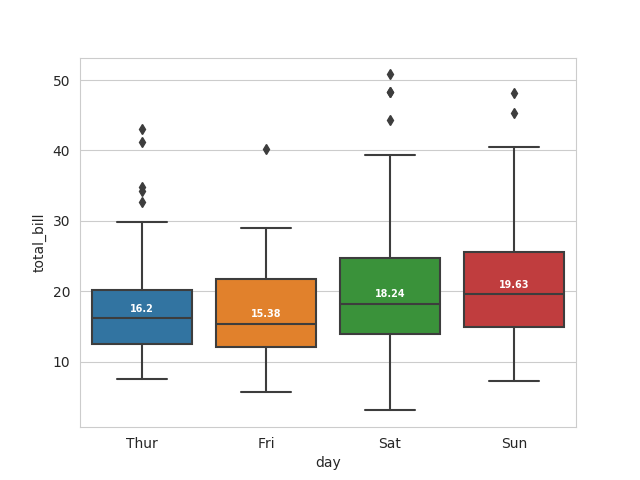Back to blog home

## Labelling Boxplot in Seaborn with Median Value

by Adam on 2016-07-29

To add another dimension to your boxplots you can label the medians with the actual value using the code below. I originally wrote code for this awhile back, and used it to answer this Stack Overflow question: https://stackoverflow.com/questions/38649501/labeling-boxplot-in-seaborn-with-median-value

``````import seaborn as sns

sns.set_style("whitegrid")
box_plot = sns.boxplot(x="day",y="total_bill",data=tips)

medians = tips.groupby(['day'])['total_bill'].median()
vertical_offset = tips['total_bill'].median() * 0.05 # offset from median

for xtick in box_plot.get_xticks():
box_plot.text(xtick,medians[xtick] + vertical_offset,medians[xtick],
horizontalalignment='center',size='x-small',color='w',weight='semibold')``````

The result is this image:tags: python seaborn median boxplot

Post thanked 0 time(s).

Related posts: